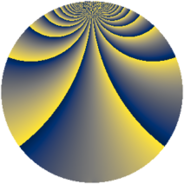# Properties

 Label 392.2.zLevel $392$ Weight $2$ Character orbit 392.z Rep. character $\chi_{392}(37,\cdot)$ Character field $\Q(\zeta_{42})$ Dimension $648$ Newform subspaces $1$ Sturm bound $112$ Trace bound $0$

# Related objects

## Defining parameters

 Level: $$N$$ $$=$$ $$392 = 2^{3} \cdot 7^{2}$$ Weight: $$k$$ $$=$$ $$2$$ Character orbit: $$[\chi]$$ $$=$$ 392.z (of order $$42$$ and degree $$12$$) Character conductor: $$\operatorname{cond}(\chi)$$ $$=$$ $$392$$ Character field: $$\Q(\zeta_{42})$$ Newform subspaces: $$1$$ Sturm bound: $$112$$ Trace bound: $$0$$

## Dimensions

The following table gives the dimensions of various subspaces of $$M_{2}(392, [\chi])$$.

Total New Old
Modular forms 696 696 0
Cusp forms 648 648 0
Eisenstein series 48 48 0

## Trace form

 $$648 q - 13 q^{2} - 13 q^{4} - 6 q^{6} - 24 q^{7} - 16 q^{8} - 76 q^{9} + O(q^{10})$$ $$648 q - 13 q^{2} - 13 q^{4} - 6 q^{6} - 24 q^{7} - 16 q^{8} - 76 q^{9} - 6 q^{10} - 47 q^{12} - 33 q^{14} - 8 q^{15} - 17 q^{16} - 26 q^{17} - 8 q^{18} - 22 q^{20} - 18 q^{22} - 26 q^{23} - 74 q^{24} - 72 q^{25} - 12 q^{26} + 2 q^{28} - 11 q^{30} + 60 q^{31} - 13 q^{32} - 14 q^{33} - 18 q^{34} + 8 q^{36} - 46 q^{38} - 32 q^{39} + 32 q^{40} - 20 q^{41} - 36 q^{42} + 38 q^{44} - 22 q^{46} - 58 q^{47} + 28 q^{48} - 16 q^{49} - 132 q^{50} + 18 q^{52} - 37 q^{54} - 32 q^{55} + 96 q^{56} - 66 q^{57} + 100 q^{60} + 28 q^{62} - 72 q^{63} - 28 q^{64} - 36 q^{65} - 4 q^{66} - 11 q^{68} - 36 q^{70} + 60 q^{71} - 130 q^{72} - 18 q^{73} - 12 q^{74} + 11 q^{76} - 132 q^{78} - 12 q^{79} - 64 q^{80} - 58 q^{81} + 152 q^{82} - 224 q^{84} + 55 q^{86} - 8 q^{87} - 169 q^{88} - 18 q^{89} + 144 q^{90} - 54 q^{92} + 154 q^{94} - 64 q^{95} - 142 q^{96} - 96 q^{97} + 151 q^{98} + O(q^{100})$$

## Decomposition of $$S_{2}^{\mathrm{new}}(392, [\chi])$$ into newform subspaces

Label Dim $A$ Field CM Traces $q$-expansion
$a_{2}$ $a_{3}$ $a_{5}$ $a_{7}$
392.2.z.a $648$ $3.130$ None $$-13$$ $$0$$ $$0$$ $$-24$$